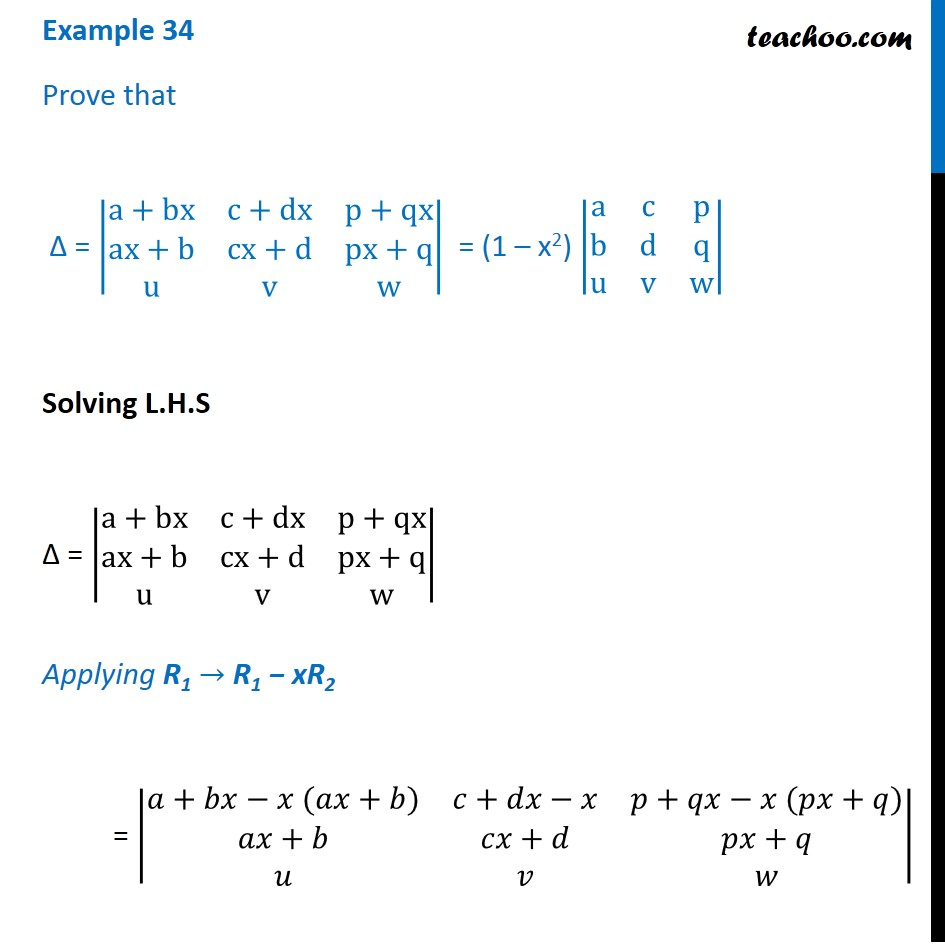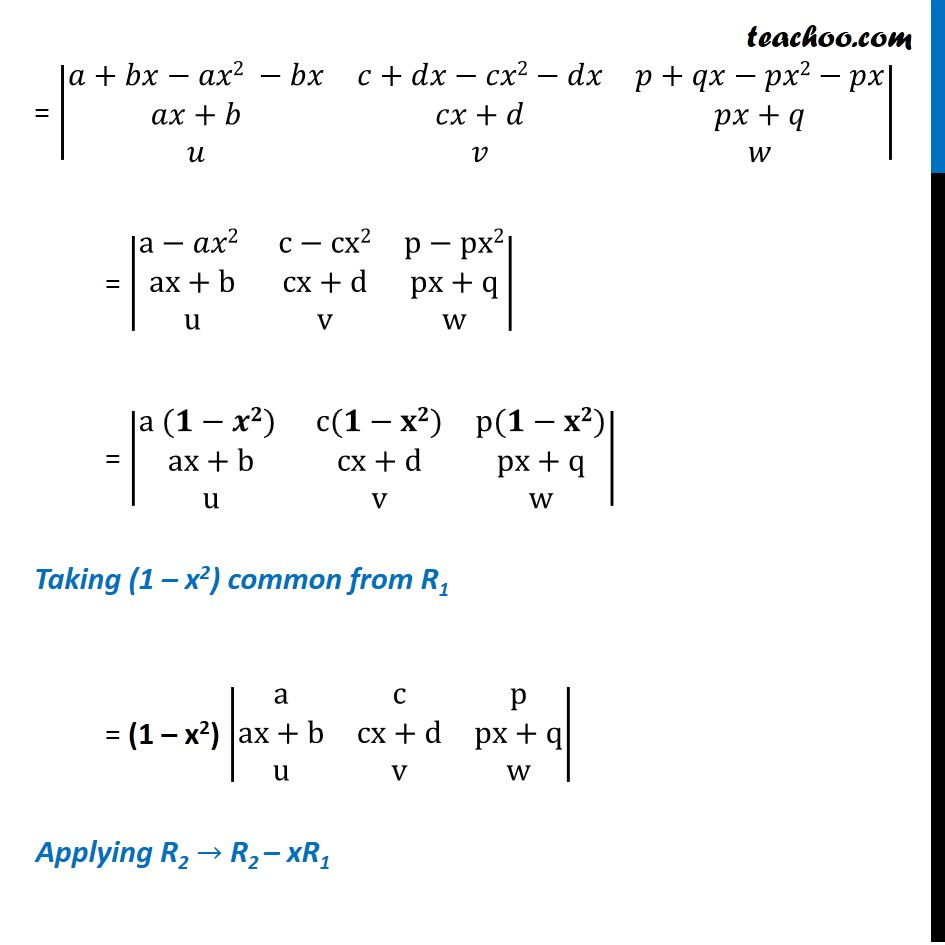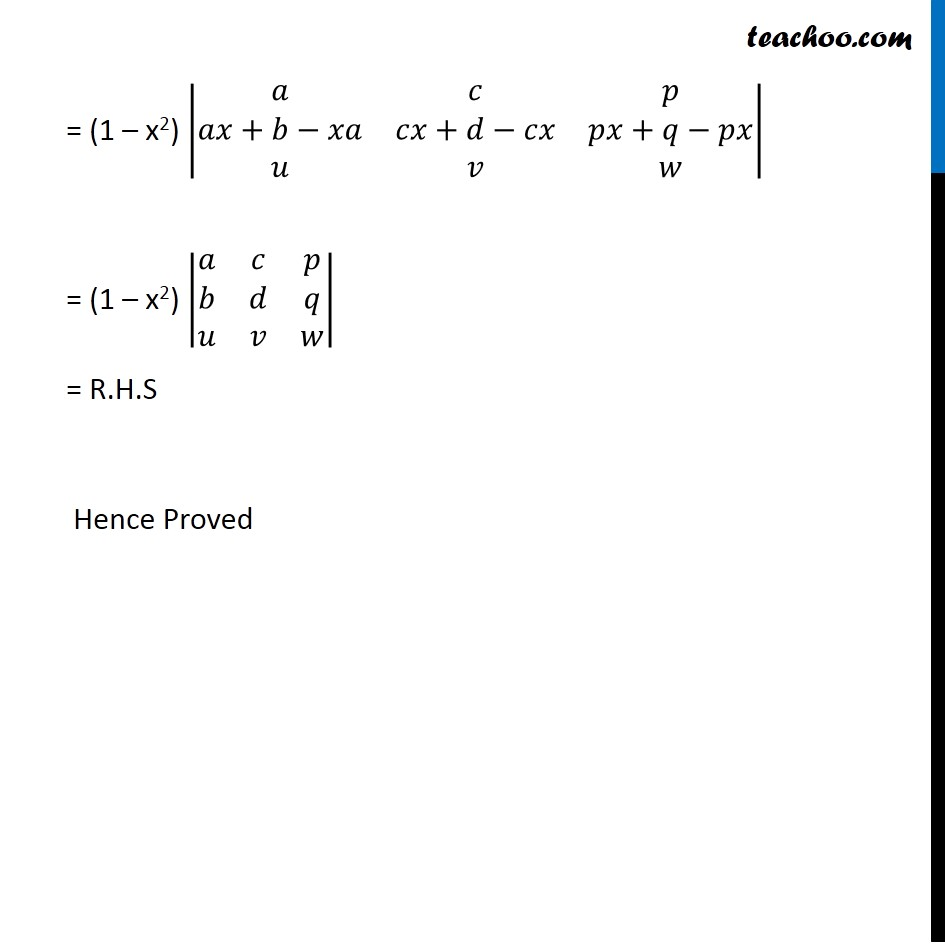Examples

Chapter 4 Class 12 Determinants (Term 1)
Serial order wise### Transcript

Example 34 Prove that Δ = |■8(a+bx&c+dx&p+qx@ax+b&cx+d&px+q@u&v&w)| = (1 – x2) |■8(a&c&p@b&d&q@u&v&w)| Solving L.H.S Δ = |■8(a+bx&c+dx&p+qx@ax+b&cx+d&px+q@u&v&w)| Applying R1 → R1 − xR2 = |■8(𝑎+𝑏𝑥−𝑥 (𝑎𝑥+𝑏)&𝑐+𝑑𝑥−𝑥&𝑝+𝑞𝑥−𝑥 (𝑝𝑥+𝑞)@𝑎𝑥+𝑏&𝑐𝑥+𝑑&𝑝𝑥+𝑞@𝑢&𝑣&𝑤)| = |■8(𝑎+𝑏𝑥−𝑎𝑥2 −𝑏𝑥&𝑐+𝑑𝑥−𝑐𝑥2−𝑑𝑥&𝑝+𝑞𝑥−𝑝𝑥2−𝑝𝑥@𝑎𝑥+𝑏&𝑐𝑥+𝑑&𝑝𝑥+𝑞@𝑢&𝑣&𝑤)| = |■8(a−𝑎𝑥2 &c−cx2&p−px2@ax+b&cx+d&px+q@u&v&w)| = |■8(a (𝟏−𝒙𝟐) &c(𝟏−𝐱𝟐)&p(𝟏−𝐱𝟐)@ax+b&cx+d&px+q@u&v&w)| Taking (1 – x2) common from R1 = (1 – x2) |■8(a&c&p@ax+b&cx+d&px+q@u&v&w)| Applying R2 → R2 – xR1 = |■8(𝑎+𝑏𝑥−𝑎𝑥2 −𝑏𝑥&𝑐+𝑑𝑥−𝑐𝑥2−𝑑𝑥&𝑝+𝑞𝑥−𝑝𝑥2−𝑝𝑥@𝑎𝑥+𝑏&𝑐𝑥+𝑑&𝑝𝑥+𝑞@𝑢&𝑣&𝑤)| = |■8(a−𝑎𝑥2 &c−cx2&p−px2@ax+b&cx+d&px+q@u&v&w)| = |■8(a (𝟏−𝒙𝟐) &c(𝟏−𝐱𝟐)&p(𝟏−𝐱𝟐)@ax+b&cx+d&px+q@u&v&w)| Taking (1 – x2) common from R1 = (1 – x2) |■8(a&c&p@ax+b&cx+d&px+q@u&v&w)| Applying R2 → R2 – xR1 = (1 – x2) |■8(𝑎&𝑐&𝑝@𝑎𝑥+𝑏−𝑥𝑎&𝑐𝑥+𝑑−𝑐𝑥&𝑝𝑥+𝑞−𝑝𝑥@𝑢&𝑣&𝑤)| = (1 – x2) |■8(𝑎&𝑐&𝑝@𝑏&𝑑&𝑞@𝑢&𝑣&𝑤)| = R.H.S Hence Proved